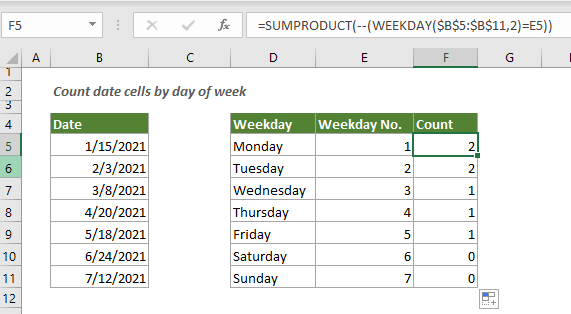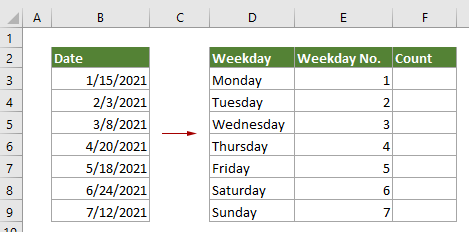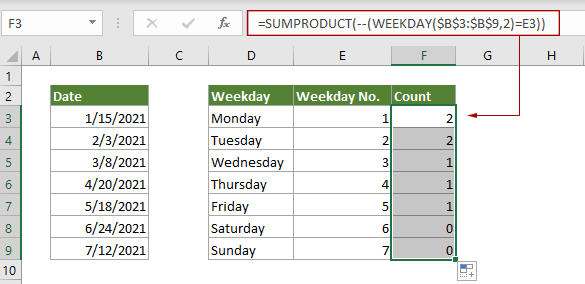## Count number of date cells in a range by day of week

Excel provides the combination of SUMPRODUCT and WEEKDAY functions to help us easily count the number of specified weekdays within a range. This tutorial provides a step by step guide to help you get through it.#### How to count date cells by day of week?

As the below screenshot shown, B3:B9 is the original data range where you want to count the dates, D3:D9 contains the sequential weekday names, and E3:E9 contains the sequential numbers from 1 to 7 to represent the weekday numbers. To count how many dates in B3:B9 equal to Monday, Tuesday, Wednesday and so on, you can do as follows.Generic Formula

=SUMPRODUCT(--(WEEKDAY(dates)=day_num))

Arguments

Dates (required): The range contains the dates we want to count.

Day_num (required): The weekday number we will count based on.

How to use this formula?

1. Select a blank cell to output the result.

2. Enter the below formula into it and press the Enter key. Select the result cell, and then drag its AutoFill Handle down to other cells in current column to apply the formula.

=SUMPRODUCT(--(WEEKDAY(\$B\$3:\$B\$9,2)=E3))Note: If there are blank cells in the original range, you will get incorrect results. To exclude blank cells, please apply the below formula:

=SUMPRODUCT((WEEKDAY(\$B\$3:\$B\$9,2)=E3)*(\$B\$3:\$B\$9<>""))

How this formula works?

=SUMPRODUCT(--(WEEKDAY(\$B\$3:\$B\$9,2)=E3))

• WEEKDAY(\$B\$3:\$B\$9,2): The WEEKDAY function returns an integer number from 1 to 7 to represent the day of week from B3:B9. Here the result is {5;3;1;2;2;4;1}.
• {5;3;1;2;2;4;1}=E3: {5;3;1;2;2;4;1}=1 Here check if each number in the array equals to 1, and return an array of TURE/FALSE values as {FALSE;FALSE;TRUE;FALSE;FALSE;FALSE;TRUE}.
• --({FALSE;FALSE;TRUE;FALSE;FALSE;FALSE;TRUE}): These two minus signs convert “TRUE” into 1 and convert “FALSE” into 0. Here you will get a new array as {0;0;1;0;0;0;1}.
• SUMPRODUCT{0;0;1;0;0;0;1}: The SUMPRODUCT function sums all number in the array and returns the final result as 2.

#### Related functions

Excel SUMPRODUCT function
The Excel SUMPRODUCT function can be used to multiply two or more columns or arrays together, and then get the sum of products.

Excel WEEKDAY function
The Excel WEEKDAY function returns an integer number from 1 to 7 to represent the day of the week for a given date in Excel.

#### Related formulas

Count cells that do not contain errors
This tutorial provides detailed steps to help you count the number of cells that do not contain errors in a specified range in Excel.

Count number of cells that do not contain many values
This post will step through how to count number of cells that do not contain many values in a specified range in Excel.

Count Cells That Do Not Contain Specific Text
You can use the COUNTIF function with a wildcard to count the number of cells that contain specific text in a range. On the contrary, it is also easy to use the COUNTIF function to count cells that do not contain specific text. This tutorial provides detailed steps to help solving the problem.

Count The Number Of Text Cells
To count the number of cells that contain text in a specified range, the COUNTIF function can help to easily get it done. Here this article will introduce the formula in details to help you solve the problem.

### The Best Office Productivity Tools

#### Kutools for Excel - Helps You To Stand Out From Crowd

 Popular Features: Find, Highlight or Identify Duplicates  |  Delete Blank Rows  |  Combine Columns or Cells without Losing Data  |  Round without Formula ... Super VLookup: Multiple Criteria  |  Multiple Value  |  Across Multi-Sheets  |  Fuzzy Lookup... Adv. Drop-down List: Easy Drop Down List  |  Dependent Drop Down List  |  Multi-select Drop Down List... Column Manager: Add a Specific Number of Columns  |  Move Columns  |  Toggle Visibility Status of Hidden Columns  |  Compare Columns to Select Same & Different Cells ... Featured Features: Grid Focus  |  Design View  |  Big Formula Bar  |  Workbook & Sheet Manager | Resource Library (Auto Text)  |  Date Picker  |  Combine Worksheets  |  Encrypt/Decrypt Cells  |  Send Emails by List  |  Super Filter  |  Special Filter (filter bold/italic/strikethrough...) ... Top 15 Toolsets:  12 Text Tools (Add Text, Remove Characters ...)  |  50+ Chart Types (Gantt Chart ...)  |  40+ Practical Formulas (Calculate age based on birthday ...)  |  19 Insertion Tools (Insert QR Code, Insert Picture from Path ...)  |  12 Conversion Tools (Numbers to Words, Currency Conversion ...)  |  7 Merge & Split Tools (Advanced Combine Rows, Split Excel Cells ...)  |  ... and more

Kutools for Excel Boasts Over 300 Features, Ensuring That What You Need is Just A Click Away...#### Office Tab - Enable Tabbed Reading and Editing in Microsoft Office (include Excel)

• One second to switch between dozens of open documents!
• Reduce hundreds of mouse clicks for you every day, say goodbye to mouse hand.
• Increases your productivity by 50% when viewing and editing multiple documents.
• Brings Efficient Tabs to Office (include Excel), Just Like Chrome, Edge and Firefox.